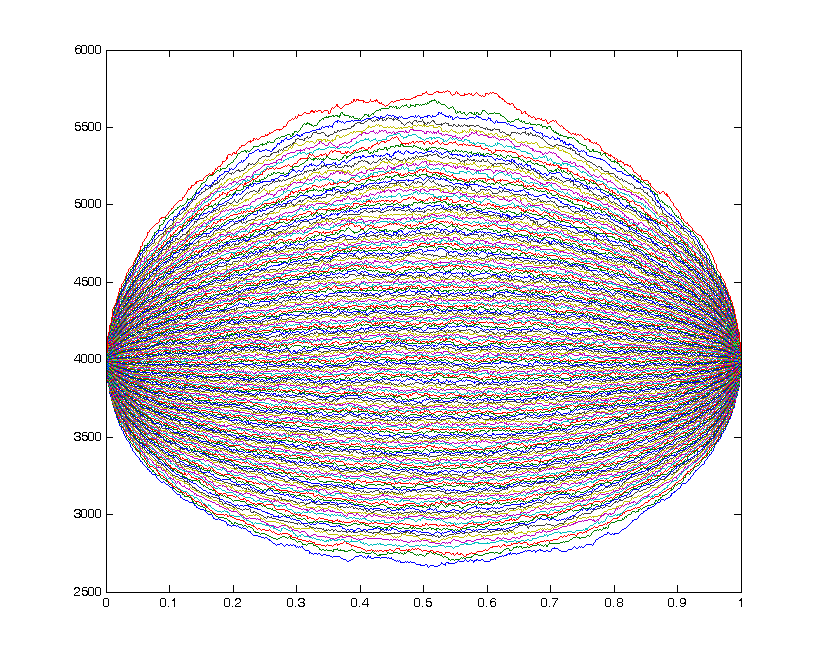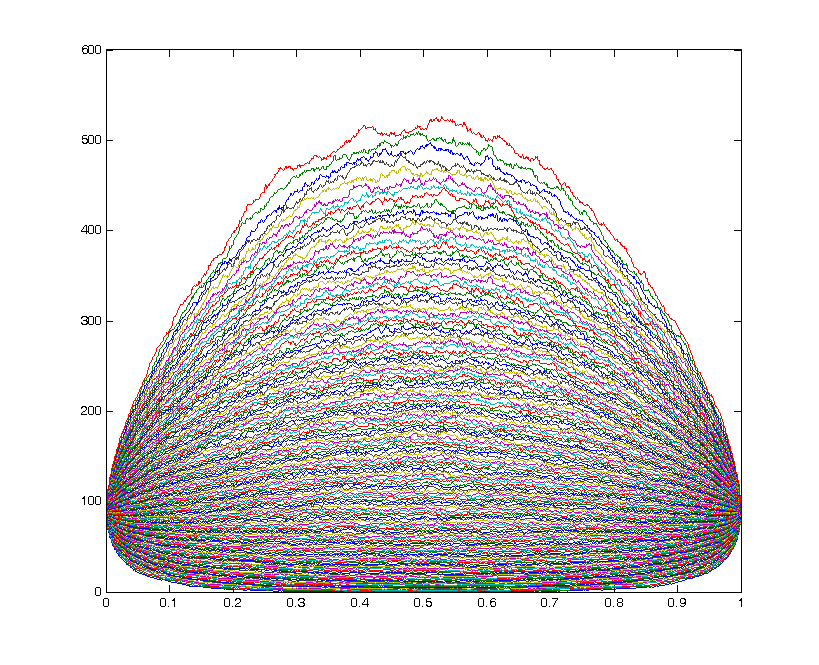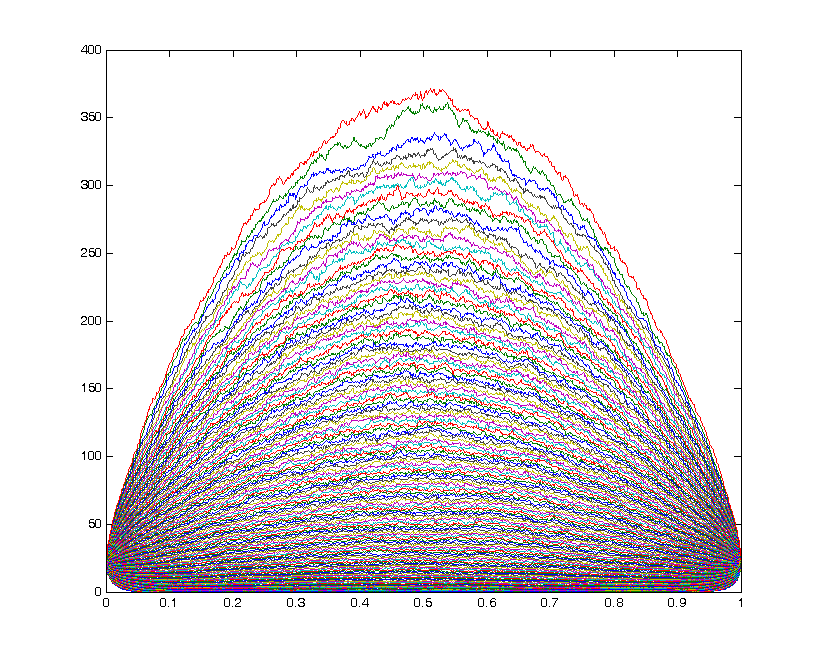###### Region of integration for matrix valued orthogonal polynomials in three variables.
arXiv:1706.01927 : Matrix elements of irreducible representations of SU(n+1)xSU(n+1) and multivariable matrix-valued orthogonal polynomials.
###### A plot of the zeros of orthogonal polynomials with respect to Bessel functions as the degree increases.
arXiv:1406.0969: Zeros of polynomials that are orthogonal with respect to Bessel weight functions.

For ν=0.25.

For ν=1.

For ν=2.

###### A video of non-intersecting squared Bessel paths.

arXiv:0911.3831 : [pdf, ps, other] Recurrence relations and vector equilibrium problems arising from a model of non-intersecting squared Bessel paths.

arXiv:1105.2481: Non-intersecting squared Bessel paths with one positive starting and ending point.

And some pictures: